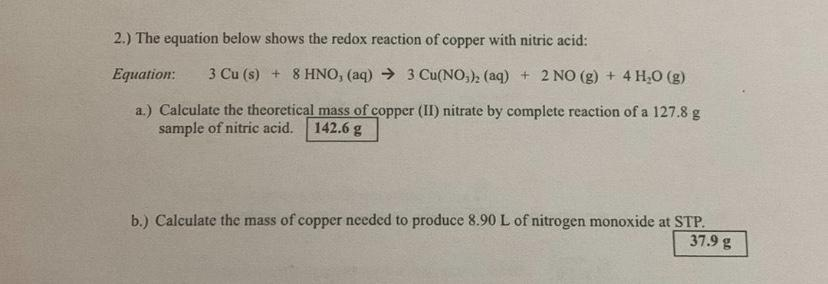Question:

# 2.) The equation below shows the redox reaction of copper with nitric acid: Equation: 3 Cu (s) + 8 HNO, (aq) → 3 Cu(NO3), (aq) +2.) The equation below shows the redox reaction of copper with nitric acid: Equation: 3 Cu (s) + 8 HNO, (aq) → 3 Cu(NO3), (aq) + 2 NO(g) + 4H2O(g) a.) Calculate the theoretical mass of copper (II) nitrate by complete reaction of a 127.8 g sample of nitric acid. 142.6 g b.) Calculate the mass of copper needed to produce 8.90 L of nitrogen monoxide at STP. 37.9 g